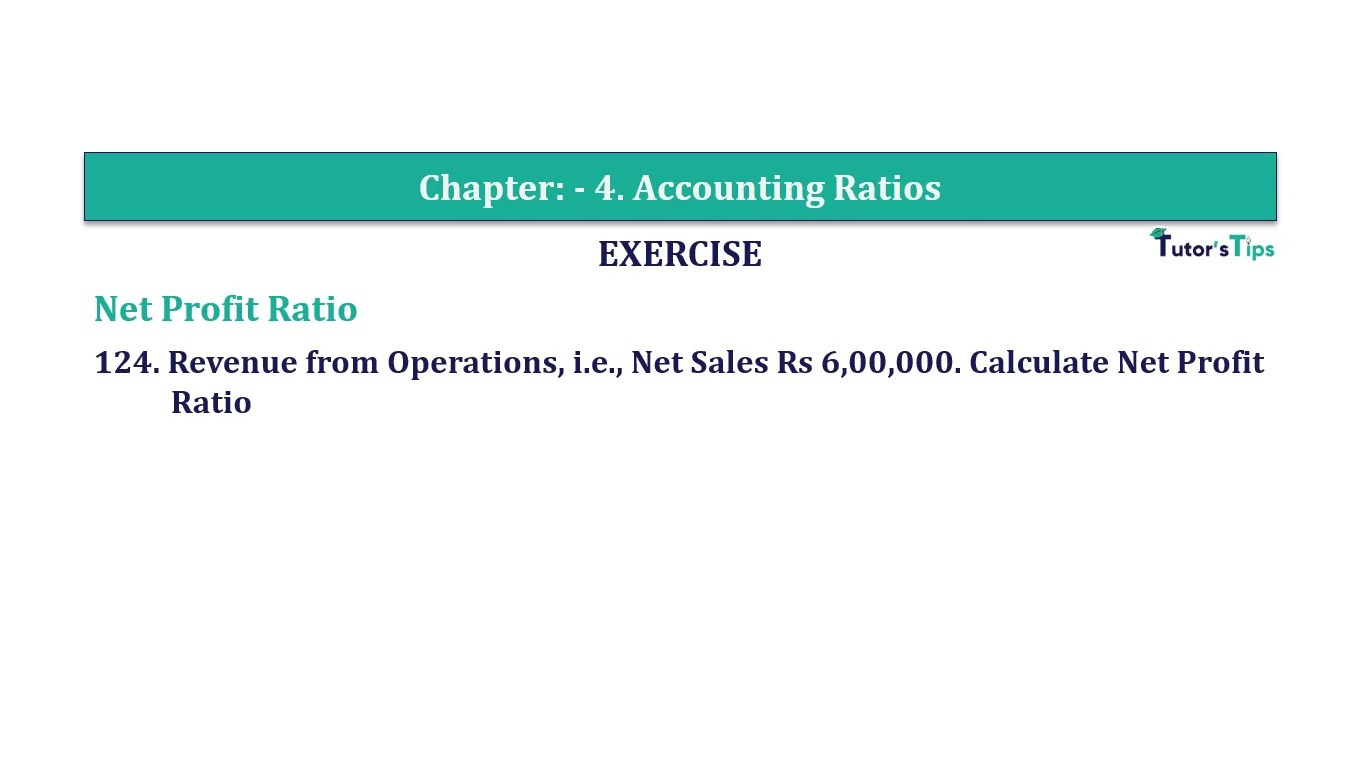# Question 124 Chapter 4 of +2-B – T.S. Grewal 12 ClassQuestion No. 124- Chapter No.4 - T.S. Grewal +2 Book Part B

Question 124 Chapter 4 of +2-B

Net Profit Ratio

124. Revenue from Operations, i.e., Net Sales Rs 6,00,000. Calculate Net Profit Ratio

### The solution of Question 124 Chapter 4 of +2-B: –

 Net Sales = Rs. 6,00,000 Net Profit = Rs. 60,000

 Net Profit Ratio = Operating Cost X 100 Net Sales
 Net Profit Ratio = Rs. 60,000 X 100 Rs. 6,00,000 = 10%

Balance Sheet: Meaning, Format & Examples

Comment if you have any question.

Also, Check out the solved question of previous Chapters: –